# Instructional Video Series Overview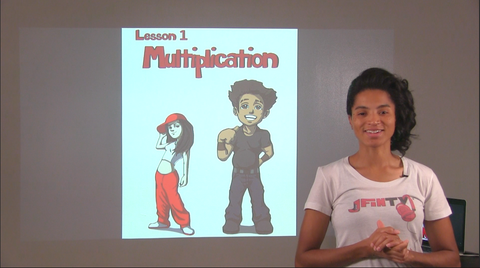Here, on our Lesson Title Page, Mrs. Finney presents the math concept.  She also introduces you to the lyrical mathematician character(s) that will join us in the word problems throughout each lesson.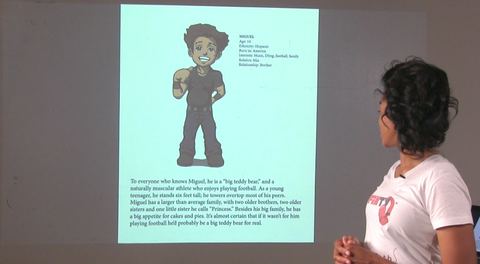On our character profile page, Mrs. Finney invites you to read the lyrical mathematician(s) profile and biography to learn more about them. As you get to know each lyrical mathematician through both the character profile page and word problems, it is likely for you to make some connections between the characters and yourself as a lyrical mathematician in the same classroom.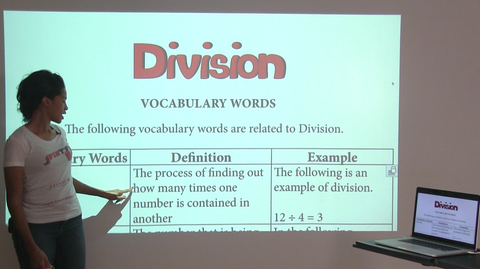To better prepare for each lesson, we begin with our vocabulary page.  Here, Mrs. Finney explains each math vocabulary word through a definition and example.  Be ready to refer to these vocabulary words as you solve your math problems and rap the lyrical math songs.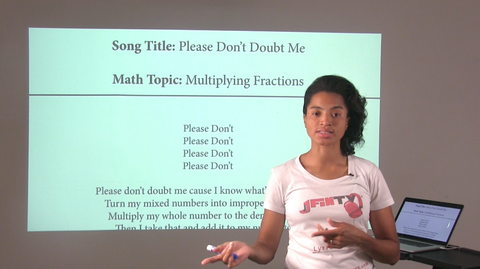On the song title page, Mrs. Finney introduces us to lyrics that explain a strategy used to solve math problems related to each lesson.  The music and visual aids are designed for edutainment and to help us recall a math strategy that we can apply to each lesson.The lyrics, math notes and examples page, provides a detailed explanation of the meaning behind each line in the song.  Mrs. Finney provides a detailed mathematical explanation and example for the lyrics in each song.  She further explains how these lyrics apply to the math strategy used in each lesson.On the procedural explanation page, Mrs. Finney provides a step-by-step explanation of a strategy used to solve the mathematical problems presented in each lesson.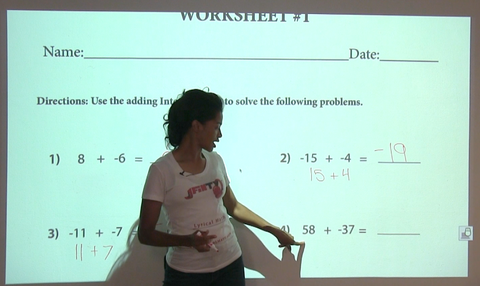To get started with applying the lyrics to mathematical problems, Mrs. Finney will complete Worksheet #1 with you!  Here, you will practice using the lyrics to solve mathematical computation problems.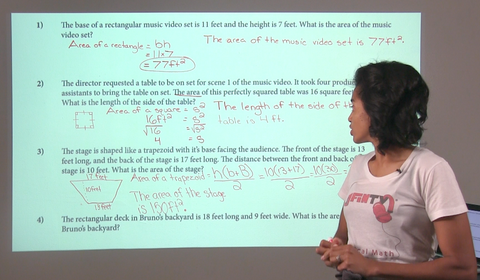Worksheet #2 consists of word problems related to the lyrical mathematician character(s) for that lesson. Mrs. Finney will guide you through the first word problem to ensure you know how to show your work and express your answer in a complete sentence.  She will then provide you with a musical timer set at three or five minutes to complete the rest of Worksheet #2 on your own. Once time is up, Mrs. Finney will review the answers with you.Worksheet #3 is a combination of both computation problems and word problems related to each lesson.  Here, Mrs. Finney will encourage you to complete Worksheet #3 on your own. During this time, the instrumental track will play to help you recall lyrics and solve the math problems. Mrs. Finney will then return to review the answers with you.Worksheet #4, the critical thinking page, will require you to solve a real-life word problem that involves the use of two or more math strategies. Mrs. Finney will read the dialogue of the lyrical mathematicians involved in the critical thinking problem and challenge you to find the solution.  A three to five-minute timer will be set. After working on your own to show your work and answer the question in a complete sentence, Mrs. Finney will join you to review your answer.